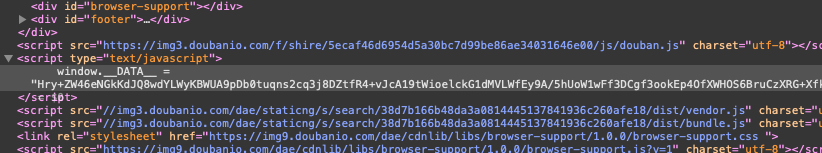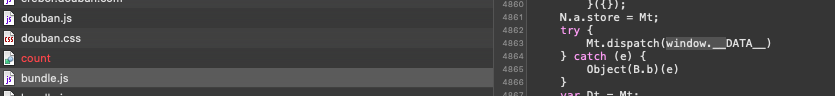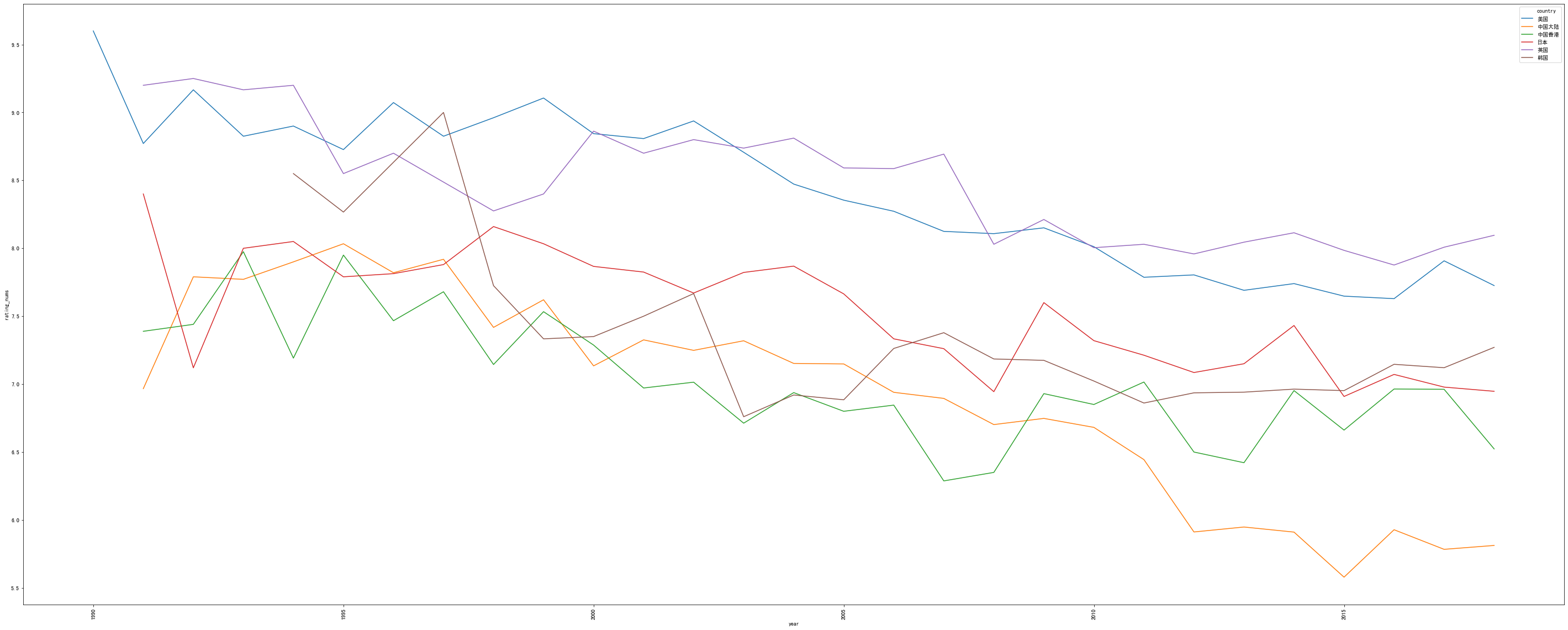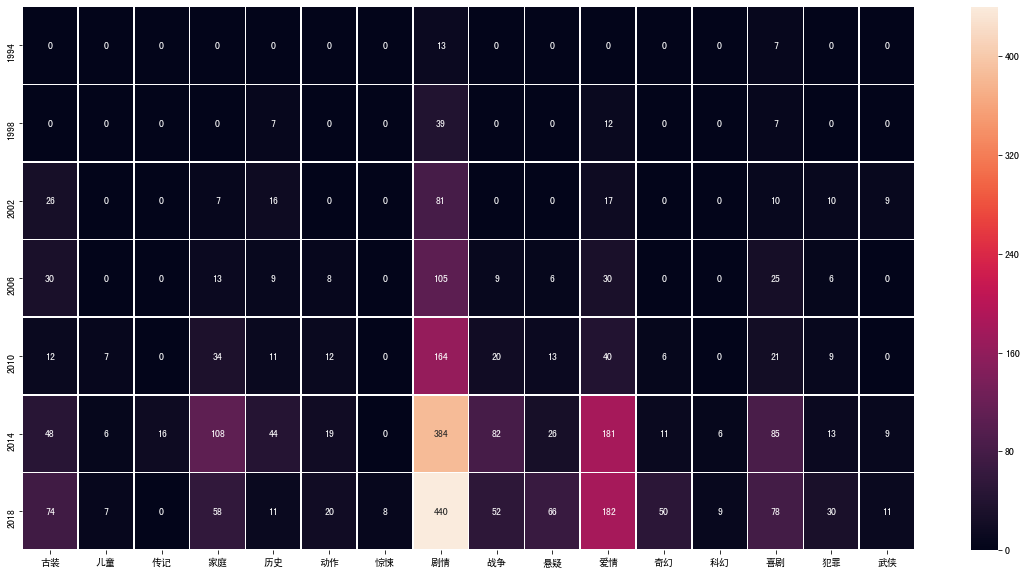## 关于 splash

Splash 是一个针对 js 的渲染服务。它内置了一个浏览器和 http 接口。基于 Python3 和 Twisted 引擎。所以可以异步处理任务。

## 安装 && 运行

``````docker pull scrapinghub/splash

docker run -p 8050:8050 -p 5023:5023 scrapinghub/splash``````

## 一个简单的 splash 应用

``````import requests
from lxml import etree

url = 'http://localhost:8050/render.html?url=https://www.toutiao.com&timeout=30&wait=0.5'
# url = 'https://www.toutiao.com'

response = requests.get(url)

tree = etree.HTML(response.text)

article_titles = tree.xpath('//div[@class="title-box"]/a/text()')

print(article_titles)``````

[‘总书记来过我们家，脱贫了还要好好干’, ‘北京2020年初雪已进城！您那儿下起来了吗？’, ‘论萌娃写作业时，求生欲有多强：爸爸我给你鼓掌’, ‘秦平：弘扬黄河文化，凝聚追梦中国精神力量’, ‘苏莱曼尼之死，全世界到底在怕什么？’, ‘观景平台，“零距离”看飞机’, ‘浙江一企业保险箱被撬，120万现金仅被偷走27万！小偷：当时想起一句“名言”……’, ‘划重点2020双闰年 网友：鼠年要多上一个月的班’, ‘若美伊全面开战，中国将再获20年发展机遇期？’]

## 开始玩耍吧~

### 尝试解决### 开始排查• 解密
• 不解密

### 我是示例

#### 数据获取

``````import requests
from urllib.parse import quote
import json
import time
import pandas as pd

# 初始化Dataframe
df = pd.DataFrame()
# 记录序号
index = 0

def get_soup(url_raw):
'''
@description: 获取指定url的数据并将其解析为soup
@param {type}
url_raw {string}
@return: BeautifulSoup的解析结果
'''
try:
## lua脚本
lua = '''
function main(splash, args)
assert(splash:go("'''+url_raw+'''"))
return {
html = splash:html(),
png = splash:png(),
har = splash:har(),
}
end
'''
url = 'http://localhost:8050/execute?lua_source=' + quote(lua)
response = requests.get(url)
soup = BeautifulSoup(js['html'])
return soup

except Exception as e:
print(e)
time.sleep(10)

def html_parser(soup,year):
'''
@description: 解析网页，提取结果
@param {type}
soup {BeautifulSoup} 解析完成的soup
year {int} 第几年
@return:
'''
tv_detail = [foo for foo in soup.find_all(class_='item-root') if re.search('.*'+str(year)+'.*',foo.find(class_='title-text').text)]
for foo in tv_detail:
index+=1
if foo.find(class_='rating_nums'):
df.loc[index,'year'] = year
df.loc[index,'rating_nums'] = foo.find(class_='rating_nums').text
df.loc[index,'rating_people'] = re.search('\D*(\d*)\D*',foo.find(class_='pl').text).group(1)
df.loc[index,'title'] = foo.find(class_='title-text').text
split_res = foo.find(class_='meta abstract').text.replace(' ','').split('/')
df.loc[index,'country'] = split_res
df.loc[index,'tv_type'] = ','.join([foo for foo in split_res[1:] if len(foo)<=2])

for year in range(1991,2019):
# url后缀参数
start_num = 0

while(1):
url_raw_1 = "https://search.douban.com/movie/subject_search?search_text={}+电视剧&start={}".format(year,start_num*15)
soup = get_soup(url_raw_1)
html_parser(soup,year)
# 判断是否存在后续页（若无，则该年结束，继续下一年的爬取）
if not soup.find(class_='next activate'):
start_num+=1
else:
# 这一过程耗时较长，为了防止意外导致数据丢失，所以每一年的爬取完成之后，保存结果
df.to_csv('tv_data_1990_2018.csv')
break
``````
``df.info()``
``````<class 'pandas.core.frame.DataFrame'>
Int64Index: 7426 entries, 3 to 8817
Data columns (total 6 columns):
year             7426 non-null float64
rating_nums      7426 non-null object
rating_people    7426 non-null object
title            7426 non-null object
country          7426 non-null object
tv_type          7426 non-null object
dtypes: float64(1), object(5)
memory usage: 726.1+ KB``````

#### 数据清洗

• 由于之前的序号不是严格顺序的（因为各种因素出现序号断层），我们将数据重新排序，并且指定新的序号（强迫症了）。
• 在上面的 info 表中，可以发现，rating_nums 和 rating_people 两项，我希望它是 float 类型的。
• 部分国家的电视剧数量太少，由于现在主要的分析目标是中国电视剧发展趋势，所以将 30 年来电视剧统计量小于 100 的剔除掉
``````import numpy as np
import matplotlib.pyplot as plt
import seaborn as sns
from collections import Counter
import re``````
``````dfnew = df.sort_index().reset_index().drop(columns=['index'])

dfnew['rating_nums'] = dfnew['rating_nums'].astype('float')
dfnew['rating_people'] = dfnew['rating_people'].astype('int')
dfnew['year'] = dfnew['year'].astype('int')

countries = [k for k,v in Counter(df.country).items() if v > 300]
df_final = dfnew.drop(index=[index for index in dfnew.index if dfnew.loc[index,'country'] not in countries])
df_final = df_final.sort_index().reset_index().drop(columns=['index'])
``````<div>
<style scoped>
.dataframe tbody tr th:only-of-type {
vertical-align: middle;
}

.dataframe tbody tr th {
vertical-align: top;
}

text-align: right;
}

</style>
<table border="1" class="dataframe">
<tr style="text-align: right;">
<th></th>
<th>year</th>
<th>rating_nums</th>
<th>rating_people</th>
<th>title</th>
<th>country</th>
<th>tv_type</th>
</tr>
<tbody>
<tr>
<th>0</th>
<td>1991</td>
<td>9.4</td>
<td>101919</td>
<td>东京爱情故事 東京ラブストーリー (1991)</td>
<td>日本</td>
<td>爱情</td>
</tr>
<tr>
<th>1</th>
<td>1991</td>
<td>9.6</td>
<td>4490</td>
<td>成长的烦恼  第七季 Growing Pains Season 7 (1991)</td>
<td>美国</td>
<td>喜剧,家庭</td>
</tr>
<tr>
<th>2</th>
<td>1991</td>
<td>9.2</td>
<td>1509</td>
<td>宋飞正传 第三季 Seinfeld Season 3 (1991)</td>
<td>美国</td>
<td>喜剧</td>
</tr>
<tr>
<th>3</th>
<td>1991</td>
<td>7.4</td>
<td>4598</td>
<td>外来妹 (1991)</td>
<td>中国大陆</td>
<td>剧情,爱情</td>
</tr>
<tr>
<th>4</th>
<td>1991</td>
<td>8.9</td>
<td>2140</td>
<td>宋飞正传 第二季 Seinfeld Season 2 (1991)</td>
<td>美国</td>
<td>喜剧</td>
</tr>
</tbody>
</table>
</div>``````

### 开始分析

``````df_year_rate = df_final[['rating_nums','year','country']].groupby(['year','country'],as_index=False).aggregate(np.average)
``````<div>
<style scoped>
.dataframe tbody tr th:only-of-type {
vertical-align: middle;
}

.dataframe tbody tr th {
vertical-align: top;
}

text-align: right;
}

</style>
<table border="1" class="dataframe">
<tr style="text-align: right;">
<th></th>
<th>year</th>
<th>country</th>
<th>rating_nums</th>
</tr>
<tbody>
<tr>
<th>0</th>
<td>1990</td>
<td>美国</td>
<td>9.600000</td>
</tr>
<tr>
<th>1</th>
<td>1991</td>
<td>中国大陆</td>
<td>6.966667</td>
</tr>
<tr>
<th>2</th>
<td>1991</td>
<td>中国香港</td>
<td>7.388889</td>
</tr>
<tr>
<th>3</th>
<td>1991</td>
<td>日本</td>
<td>8.400000</td>
</tr>
<tr>
<th>4</th>
<td>1991</td>
<td>美国</td>
<td>8.771429</td>
</tr>
</tbody>
</table>
</div>``````
``````plt.figure(figsize=(50,20))
sns.lineplot(x=df_year_rate.year,y=df_year_rate.rating_nums,hue=df_year_rate.country)
plt.xticks(rotation=90)``````
``````(array([1985., 1990., 1995., 2000., 2005., 2010., 2015., 2020.]),
<a list of 8 Text xticklabel objects>)````````````def ten_year_type_count(dften):
s1= ','.join(dften.tv_type)
all_type = re.sub(r',+',',',s1)
return [foo for foo in sorted(Counter(all_type.split(',')).items(),key=lambda item:item,reverse=True) if foo>5]

five_list = [ten_year_type_count(df_final[df_final.country=="中国大陆"][df_final.year<=foo][df_final.year>foo-4]) for foo in range(1994,2021,4)]
``````
``````type_list =list(set([foo1 for foo in five_list for foo1 in foo]))
df_five_type = pd.DataFrame(index = [1994,1998,2002,2006,2010,2014,2018],columns=type_list,data=0)
for index,five in enumerate(five_list):
for foo in five:
df_five_type.loc[1994+index*4,foo] = foo
df_five_type``````
``````<div>
<style scoped>
.dataframe tbody tr th:only-of-type {
vertical-align: middle;
}

.dataframe tbody tr th {
vertical-align: top;
}

text-align: right;
}

</style>
<table border="1" class="dataframe">
<tr style="text-align: right;">
<th></th>
<th>古装</th>
<th>儿童</th>
<th>传记</th>
<th>家庭</th>
<th>历史</th>
<th>动作</th>
<th>惊悚</th>
<th>剧情</th>
<th>战争</th>
<th>悬疑</th>
<th>爱情</th>
<th>奇幻</th>
<th>科幻</th>
<th>喜剧</th>
<th>犯罪</th>
<th>武侠</th>
</tr>
<tbody>
<tr>
<th>1994</th>
<td>0</td>
<td>0</td>
<td>0</td>
<td>0</td>
<td>0</td>
<td>0</td>
<td>0</td>
<td>13</td>
<td>0</td>
<td>0</td>
<td>0</td>
<td>0</td>
<td>0</td>
<td>7</td>
<td>0</td>
<td>0</td>
</tr>
<tr>
<th>1998</th>
<td>0</td>
<td>0</td>
<td>0</td>
<td>0</td>
<td>7</td>
<td>0</td>
<td>0</td>
<td>39</td>
<td>0</td>
<td>0</td>
<td>12</td>
<td>0</td>
<td>0</td>
<td>7</td>
<td>0</td>
<td>0</td>
</tr>
<tr>
<th>2002</th>
<td>26</td>
<td>0</td>
<td>0</td>
<td>7</td>
<td>16</td>
<td>0</td>
<td>0</td>
<td>81</td>
<td>0</td>
<td>0</td>
<td>17</td>
<td>0</td>
<td>0</td>
<td>10</td>
<td>10</td>
<td>9</td>
</tr>
<tr>
<th>2006</th>
<td>30</td>
<td>0</td>
<td>0</td>
<td>13</td>
<td>9</td>
<td>8</td>
<td>0</td>
<td>105</td>
<td>9</td>
<td>6</td>
<td>30</td>
<td>0</td>
<td>0</td>
<td>25</td>
<td>6</td>
<td>0</td>
</tr>
<tr>
<th>2010</th>
<td>12</td>
<td>7</td>
<td>0</td>
<td>34</td>
<td>11</td>
<td>12</td>
<td>0</td>
<td>164</td>
<td>20</td>
<td>13</td>
<td>40</td>
<td>6</td>
<td>0</td>
<td>21</td>
<td>9</td>
<td>0</td>
</tr>
<tr>
<th>2014</th>
<td>48</td>
<td>6</td>
<td>16</td>
<td>108</td>
<td>44</td>
<td>19</td>
<td>0</td>
<td>384</td>
<td>82</td>
<td>26</td>
<td>181</td>
<td>11</td>
<td>6</td>
<td>85</td>
<td>13</td>
<td>9</td>
</tr>
<tr>
<th>2018</th>
<td>74</td>
<td>7</td>
<td>0</td>
<td>58</td>
<td>11</td>
<td>20</td>
<td>8</td>
<td>440</td>
<td>52</td>
<td>66</td>
<td>182</td>
<td>50</td>
<td>9</td>
<td>78</td>
<td>30</td>
<td>11</td>
</tr>
</tbody>
</table>
</div>``````
``````plt.figure(figsize=(20,10))
sns.heatmap(data=df_five_type, annot=True, fmt="d", linewidths=.5)``````
``<matplotlib.axes._subplots.AxesSubplot at 0x1a2ffe5be0>``## 总结

10 年以前，战争剧稀少，诸如《亮剑》、《团长》等优质的抗战剧大多诞生于这个时期。10 年以后，由于政策的扶持和被优质片打开的市场，战争题材的电视剧开始出现井喷，说好听点良莠不齐，说难听点，一堆杂草群魔乱舞，诸多的“神剧”就是这之后的产物。

## 后记

• 为了这一章特地看了一点 lua
• 相见时难别亦难

– 2020 年 01 月 13 日，写于夜深人静之时。